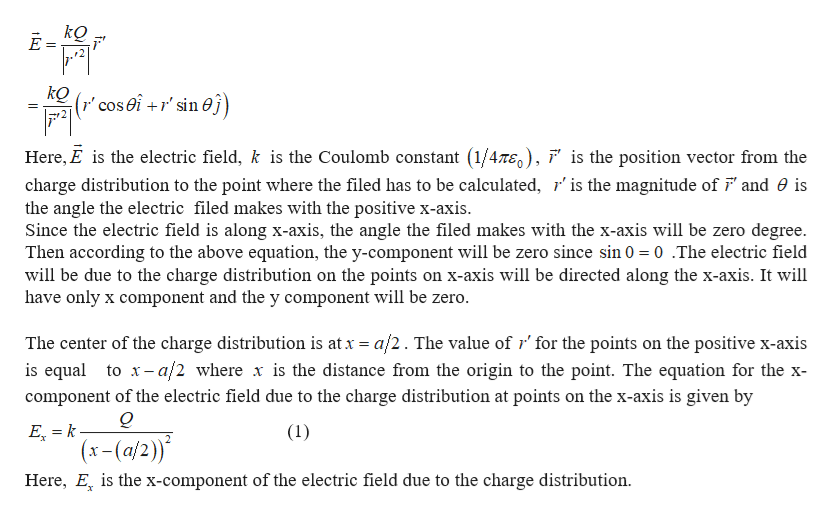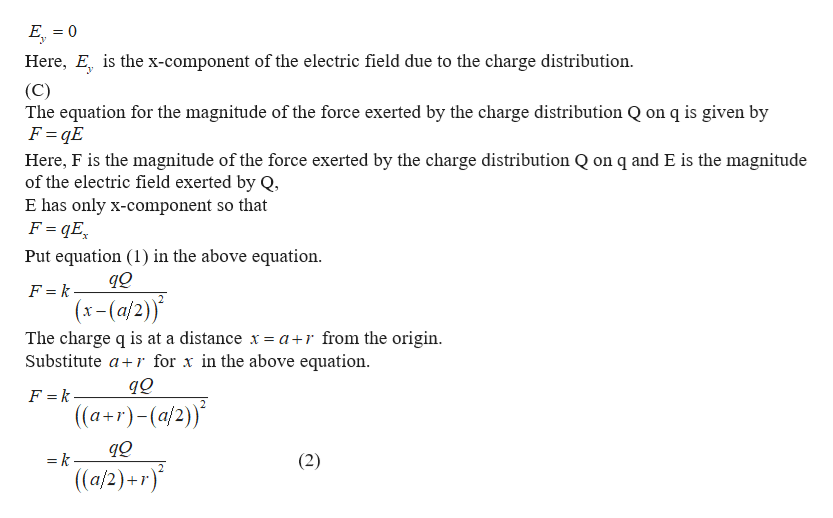yx+

Question

Positive charge Q is distributed uniformly along the x-axis from x=0 to x=a. A positive point charge q is located on the positive x-axis at x=a+r, a distance r to the right of the end of Q. (Figure 1)

Part A
Calculate the x-component of the electric field produced by the charge distribution Q
at points on the positive x-axis where x>a.
Express your answer in terms of the variables
Q, a, x, r, and appropriate constants.

Part B
Calculate the y-component of the electric field produced by the charge distribution Q at points on the positive x-axis where x>a.
Express your answer in terms of the variables
Q, a, x, r, and appropriate constants.

Part C
Calculate the magnitude of the force that the charge distribution Q exerts on q.
Express your answer in terms of the variables Q.

Part D
In what direction the charge distribution Q exerts force on q.
In what direction the charge distribution exerts force on .to the left to the right

Step 1

(A)

The equation for the electric field due to the charge distribution is given byhelp_outlineImage TranscriptionclosekQ r" cos0i +r' sin0j) Here, E is the electric field, k is the Coulomb constant (1/47E0), ' is the position vector from the charge distribution to the point where the filed has to be calculated, r' is the magnitude of F and 0 is the angle the electric filed makes with the positive x-axis Since the electric field is along x-axis, the angle the filed makes with the x-axis will be zero deg Then according to the above equation, the y-component will be zero since sin 0 0.The electric field will be due to the charge distribution on the points on x-axis will be directed along the x-axis. It will have only x component and the y component will be zero. e. The center of the charge distribution is at x a/2. The value of r' for the points on the positive x-axis is equal to x-a/2 where x is the distance from the origin to the point. The equation for the x component of the electric field due to the charge distribution at points on the x-axis is given by Q E k- (x-(a/2) Here, E is the x-component of the electric field due to the charge distribution. (1) fullscreen
Step 2

B)The equation for the y-component of the electric field due to the charge distribution at points on the x-axis is given byhelp_outlineImage TranscriptioncloseE0 Here, E is the x-component of the electric field due to the charge distribution (C) The equation for the magnitude of the force exerted by the charge distribution Q on q is given by F qE Here, F is the magnitude of the force exerted by the charge distribution Q on q and E is the magnitude of the electric field exerted by Q, E has only x-component so that F qE Put equation (1) in the above equation F = k (x-(a/2)) The charge q is at a distance x = a r from the origin Substitute ar for x in the above equation. F =k. ((a+r)-(a/2)) = k (2) (a/2)+ r) fullscreen
Step 3

(D)Since the charge distribution Q and the charge q are positive, the force between them will be repulsive. The...

Want to see the full answer?

See Solution

Want to see this answer and more?

Our solutions are written by experts, many with advanced degrees, and available 24/7

See Solution
Tagged in

Electric Charges and Fields